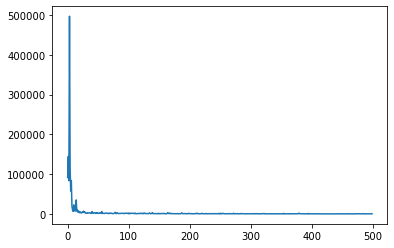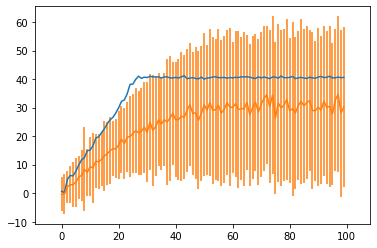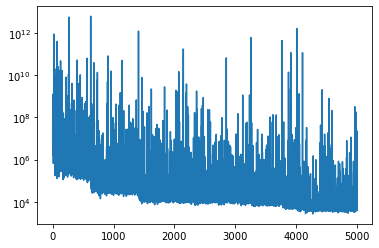# Piecewise regression with TraceEnum_ELBO

Hello Pyro experts,

I’m trying to implement a piecewise linear regression model. I’ve tried various variations on the model, but I haven’t been able to get good results. My approach is to sample change points from a Categorical and use a MaskedMixture with a linear regression as one component distribution, and another MaskedMixture as the other component (i.e. the MaskedMixture’s are nested as many times as there are pieces).

``````def piecewise_regression(x, y, n_pieces = 3):
"""
piecewise regression where pieces are connected and the last piece has slope 0
"""
N = x.shape
lines = []
change_points = []
line_distributions = []
for piece in pyro.plate('pieces', n_pieces):
if piece == 0:
lines.append(sample_linear_regression())
line_distributions.append(dist.Normal(lines[-1]*x+lines[-1],lines[-1]))
change_point = sample_change_point(x, piece)
change_points.append(change_point)
elif piece < n_pieces-1:
slope, noise_std = sample_slope_and_noise_std(piece)
prev_slope = lines[-1]
prev_intercept = lines[-1]
intercept = (prev_slope*x[change_point]+prev_intercept)-slope*x[change_point]
lines.append((slope,intercept,noise_std))
prev_dist = line_distributions[-1]
# if all of x is masked, sample_change_point returns the last x
change_point = sample_change_point(x, piece)
change_point = min(change_point + change_points[-1], N)
change_points.append(change_point)
else:
noise_std = pyro.sample('noise_std_{}'.format(piece), dist.LogNormal(0.,1.))
intercept = lines[-1]*x[change_points[-1]]+lines[-1]
lines.append((intercept,noise_std))
prev_dist = line_distributions[-1]
with pyro.plate('N', N):
y = pyro.sample("obs", line_distributions[-1], obs=y)
return y, lines, change_points, line_distributions

def sample_change_point(x, piece, mask = None):
n_iter = x.shape
change_point_probs_prior = torch.ones(n_iter)
else:
return n_iter-1
change_point = pyro.sample('change_point_{}'.format(piece), dist.Categorical(probs=change_point_probs_prior),infer={'enumerate': 'parallel'})
return change_point

def sample_linear_regression():
slope = pyro.sample('slope', dist.Normal(0.,1.))
intercept = pyro.sample('intercept', dist.Normal(0.,1.))
noise_std = pyro.sample('noise_std', dist.LogNormal(0.,1.))
return slope, intercept, noise_std

def sample_slope_and_noise_std(piece):
slope = pyro.sample('slope_{}'.format(piece), dist.Normal(0.,1.))
noise_std = pyro.sample('noise_std_{}'.format(piece), dist.LogNormal(0.,1.))
return slope, noise_std
``````

The guide has all the same distributions as the model: https://pastebin.com/sYmCpR6B

I want to enumerate the change points out with TraceEnum_ELBO, though it looks like that won’t scale very well with more change points. But even with just one change point, I don’t get a good fit, even though the elbo converges:

``````pyro.clear_param_store()
elbo = TraceEnum_ELBO(max_plate_nesting=1, num_particles=10)
svi = SVI(piecewise_regression, piecewise_regressionGuide, optim, loss=elbo)

losses = []
for i in range(500):
losses.append(svi.step(x,y,2))

plt.plot(losses)
````````````pred = pyro.infer.predictive.Predictive(pyro.poutine.uncondition(piecewise_regression),guide=piecewise_regressionGuide,num_samples=100)

fit = pred(x,y,2)

fit_obs_mean = fit['obs'].mean(0).detach().numpy()
fit_obs_std = fit['obs'].std(0).detach().numpy()

%matplotlib inline
plt.plot(x,y)
#plt.plot(x,slope_fit*x.numpy()+intercept_fit)
#plt.plot(x,fit_obs)
plt.errorbar(x,fit_obs_mean,yerr=fit_obs_std)
plt.axis('equal');
``````What can I do to make the fit more accurate? Is there a better way to implement this model? I’ve tried having Dirichlet priors on the change points, and multiple restarts (which helps a little, but best of 10 restarts still isn’t great).
Thanks

Hi @deoxy,
I’m not sure why your discrete model fails to converge, but I would guess a continuous parameterization would converge faster and more reliably, and would additionally scale to multiple pieces. Here’s an attempt at a continuous parameterization:

``````def piecewise_eval(knot_x, knot_y, x):
# I haven't unit tested this:
n_knots = knot_x.size(-1)
assert n_knots >= 2
knot_x, idx = knot_x.sort(dim=-1)
knot_y = knot_y[..., idx] # this might not play well with batching
lb = (x.unsqueeze(-1) > knot_x.unsqueeze(-2)).long().sum(-1)
lb[lb >= knot_x.size - 1] = knot_x.size - 2
ub = lb + 1
x0 = knot_x[..., lb]
x1 = knot_x[..., ub]
y0 = knot_y[..., lb]
y1 = knot_y[..., ub]
return (y1 * (x - x0) + y0 * (x1 - x)) / (x1 - x0).clamp(min=1e-8)

def piecewise_regression(x, y, n_pieces=3):
knot_x = pyro.sample("knot_x",
dist.Uniform(0, 100)
.expand([n_pieces]).to_event(n_pieces))
knot_y = pyro.sample("knot_y",
dist.Normal(0, 100)
.expand([n_pieces]).to_event(n_pieces))
y_pred = piecewise_eval(knot_x, knot_y, x)
y_scale = pyro.sample("y_scale", dist.LogNormal(0, 5))
pyro.sample("obs", dist.Normal(y_pred, y_scale),
obs=y)

guide = AutoNormal(piecwise_regression)
``````

``````def piecewise_eval(knot_x, knot_y, x):
# I haven't unit tested this:
n_knots = knot_x.size(-1)
assert n_knots >= 2
knot_x, idx = knot_x.sort(dim=-1)
knot_y = knot_y.gather(-1,idx)
lb = (x.unsqueeze(-1) > knot_x[...,1:].unsqueeze(-2)).long().sum(-1)
lb[lb >= n_knots - 1] = n_knots - 2
ub = lb + 1
x0 = knot_x.gather(-1,lb)
x1 = knot_x.gather(-1,ub)
y0 = knot_y.gather(-1,lb)
y1 = knot_y.gather(-1,ub)
slopes = (y1-y0)/(x1-x0).clamp(min=1e-8)
return slopes*x + (y1 - slopes*x1)

def piecewise_regression(x, y, n_pieces=3):
knot_x = pyro.sample("knot_x",
dist.Uniform(0, 100)
.expand([n_pieces]).to_event(0))
knot_y = pyro.sample("knot_y",
dist.Normal(0, 100)
.expand([n_pieces]).to_event(0))
y_pred = piecewise_eval(knot_x, knot_y, x)
y_scale = pyro.sample("y_scale", dist.LogNormal(0, 1))
y = pyro.sample("obs", dist.Normal(y_pred, y_scale),
obs=y)
return y
``````

and train with an AutoDiagonalNormal guide. It seems like the parameters basically remain close to the initialization, no matter the learning rate and number of iterations (see notebook).
I know it’s not a lot to go on, but any ideas on what I could do to make it work?

is that `clamp` what you want? maybe it’s blocking all gradient flow?

I tried removing the clamp, but with or without it the gradient norms are big:(that’s the AutoDiagonalNormal loc gradient)
I did get a good fit once with a different version of the model and a custom guide, which I’m guessing was due to a lucky initialization, on n_pieces = 2 (i.e. just regular linear regression) data. It’s strange if a different parametrization should make it that much harder.

I changed the model so that the knot parameters are sampled from U(0,1) and N(0,1) and then scaled by the length of x, and it works now. I updated the notebook above in case anyone is interested. Thanks for the help @fritzo, @martinjankowiak!

1 Like

Nice notebook @deoxy! If you ever feel like turning that into a tutorial we’d be happy to host that at https://pyro.ai/examples, and I’d be happy to review